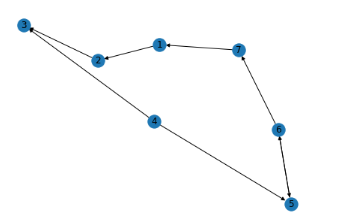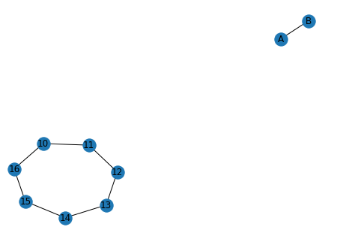# Finding the mother vertex in a graph

Networks or graphs are pivotal in so many real-world applications such as fraud prevention systems. search engines, recommendation systems, social networks and a lot more. The search for the mother vertex of a graph aids in understanding the accessibility of a given vertex or collection of vertices.

What is the meaning of the mother vertex in a given graph?

In a given graph G = (V, E), a mother vertex v has a pathway for all other vertices in the specified graph to enable the connection. All other vertices in the graph can be accessed via the mother vertex. A mother vertex is quite common in directed graphs but is also applicable in undirected networks. We will briefly explore mother vertices in different network examples

Directed graph: Directed graphs or DiGraphs do hold directed edges or nodes. These edges can be unidirectional or bidirectional. For DiGraphs, self-loops are permissible but parallel edges are not. Mother vertex exists in directed graphs and there can be multiple of these as shown below.  Based on the directed graph below, nodes  and  are the mother vertex.Undirected connected graph: A mother vertex can be present on undirected connected graphs. In undirected graphs, self-loops are allowed while parallel edges are not permitted. The undirected connected graph below displays nodes 11 to 16 connected by seven non-directional edges. In this type of undirected connected graph, every node is considered to be a mother vertex.

Undirected disconnected graph: There is no mother vertex in the example graph below. This is because no edge connects node  to node [A]. It is safe to say that undirected disconnected graphs do not have mother vertex.Python implementation of finding a mother vertex

We will run through a mother vertex implementation in Python. The first code example returns no mother vertex from a selection of 12 nodes.

``````from collections import defaultdict

# A cass of a directed graph using adjacency list

class Graph:

def __init__(self,vertices):
self.V = vertices #No. of vertices
self.graph = defaultdict(list) # default dictionary

# DFS traversing from v
def DFSUtil(self, v, visited):

# Idenifying the current node as visited and print it
visited[v] = True

# Noting all the vertices adjacent to this vertex
for i in self.graph[v]:
if visited[i] == False:
self.DFSUtil(i, visited)

# Now adding w to the list of v
self.graph[v].append(w)

# If a mother vertex exists return. Otherwise returns -1
def findMother(self):

visited =[False]*(self.V)

# Do a DFS traversal and find the last finished
# vertex
for i in range(self.V):
if visited[i]==False:
self.DFSUtil(i,visited)
v = i

# If there exist mother vertex (or vetices) in given
# graph, then v must be one (or one of them)

# Now check if v is actually a mother vertex (or graph
# has a mother vertex). We basically check if every vertex
# is reachable from v or not.

# Reset all values in visited[] as false and do
# DFS beginning from v to check if all vertices are
# reachable from it or not.
visited = [False]*(self.V)
self.DFSUtil(v, visited)
if any(i == False for i in visited):
return "Not Present"
else:
return v

# Create a graph given in the above diagram
g = Graph(12)
print ("A mother vertex is " + str(g.findMother()))

A mother vertex is Not Present``````

On the other hand, the example below identifies node  as the mother vertex in the below graph

``````from collections import defaultdict

# A cass of a directed graph using adjacency list

class Graph:

def __init__(self,vertices):
self.V = vertices #No. of vertices
self.graph = defaultdict(list) # default dictionary

# DFS traversing from v
def DFSUtil(self, v, visited):

# Idenifying the current node as visited and print it
visited[v] = True

# Noting all the vertices adjacent to this vertex
for i in self.graph[v]:
if visited[i] == False:
self.DFSUtil(i, visited)

# Now adding w to the list of v
self.graph[v].append(w)

# If a mother vertex exists return. Otherwise returns -1
def findMother(self):

visited =[False]*(self.V)

# Do a DFS traversal and find the last finished
# vertex
for i in range(self.V):
if visited[i]==False:
self.DFSUtil(i,visited)
v = i

# If there exist mother vertex (or vetices) in given
# graph, then v must be one (or one of them)

# Now check if v is actually a mother vertex (or graph
# has a mother vertex). We basically check if every vertex
# is reachable from v or not.

# Reset all values in visited[] as false and do
# DFS beginning from v to check if all vertices are
# reachable from it or not.
visited = [False]*(self.V)
self.DFSUtil(v, visited)
if any(i == False for i in visited):
return "Not Present"
else:
return v

# Create a graph given in the above diagram
g = Graph(12)